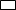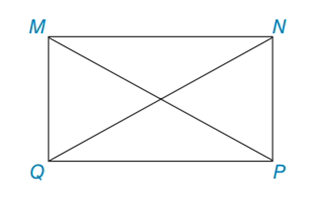Chapter 4.3, Problem 13EElementary Geometry For College St...

7th Edition
Alexander + 2 others
ISBN: 9781337614085

Solutions

Chapter
SectionElementary Geometry For College St...

7th Edition
Alexander + 2 others
ISBN: 9781337614085
Textbook ProblemIn Exercises 11 to 14, consider MNPQ with diagonals M P ¯  and  N Q ¯ . When the answer is not a whole number, leave a square root answer.Exercises 11 – 14If NP = 7 and MP = 11, find QP and MN.

To determine

To find:

QP and MN of the given rectangle.

Explanation

The basic property of rectangle:

1) All the properties of a parallelogram apply (the ones that matter here are parallel sides, opposite sides are congruent, and diagonals bisect each other).

2) All angles are right angles by definition.

3) The diagonals are congruent.

Calculation:

Given: NP = 7 and MP = 11

Consider the rectangle.

To find QP and MN:

Let us take right MNP.

By using Pythagorean Theorem

MP2=NP2+MN2112=72+M

Still sussing out bartleby?

Check out a sample textbook solution.

See a sample solution

The Solution to Your Study Problems

Bartleby provides explanations to thousands of textbook problems written by our experts, many with advanced degrees!

Get Started

In Exercises 107-120, factor each expression completely. 111. 10 14x 12x2

Applied Calculus for the Managerial, Life, and Social Sciences: A Brief Approach

For the following scores, find the value of each expression: X 3 2 4 2 a. X b. (X)2 c. X 2 d. (X 2)

Essentials of Statistics for The Behavioral Sciences (MindTap Course List)

The third partial derivative wxyz of the function w=4xyz+x3y2z+x3 .

Mathematical Applications for the Management, Life, and Social Sciences

Subtract: 8yd1ft3in.2yd2ft6in._

Elementary Technical Mathematics

Differentiate the function. h(u)=Au3+Bu2+Cu

Single Variable Calculus: Early Transcendentals

True or False: is monotonic.

Study Guide for Stewart's Single Variable Calculus: Early Transcendentals, 8th